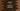# Python in and not in operators explanation with examples## Python in and not in operators:

Both in and not in operators are used to check if an item is in a sequence or not in Python. In this post, we will learn how to use in and not in operators in Python with examples.

### Python in operator:

Python in operator is used to check if an item is in a sequence or not. This operator returns a boolean value. So, if the element is in that sequence, it returns True, else it returns False.

We can use it with sequences like array, list, tuple, list etc.

Let me show you examples with different types of sequences.

#### Example of in operator with array:

Let’s try it with arrays:

``````given_arr = [1,2,3,4,5]

print(1 in given_arr)

print(10 in given_arr)``````

For this example, given_arr is the array and we are checking 1 and 10 is in that array or not by using the in operator.

If you run this program, it will print:

``````True
False``````

The first line prints True because 1 is in given_arr. The second line prints False because 10 is not in given_arr.

Let’s take the number as input from the user and check if it is in given_arr or not.

``````given_arr = [1,2,3,4,5]

i = int(input('Enter a number: '))

if i in given_arr:
print(f'{i} is in {given_arr}')
else:
print(f'{i} is not in {given_arr}')``````

The number we are taking as input is stored in i. The if-else statement checks if that number is in given_arr or not. Based on its return value, it prints a message.

It will give output as like below:

``````Enter a number: 3
3 is in [1, 2, 3, 4, 5]

Enter a number: 10
10 is not in [1, 2, 3, 4, 5]``````

#### Python in operator example with a tuple:

Let’s try it with a tuple:

``````given_tuple = ('sun', 'mon', 'tues', 'wed', 'thurs')

word = input('Enter a word: ')

if word in given_tuple:
print(f'{word} is in {given_tuple}')
else:
print(f'{word} is not in {given_tuple}')``````

In this example, given_tuple is a tuple of words. It takes a word as input and stores that in the word variable.

The if-else statement checks if word is in given_tuple or not and based on the return boolean, it prints one message.

It will give output as like below:

``````Enter a word: hello
hello is not in ('sun', 'mon', 'tues', 'wed', 'thurs')

Enter a word: wed
wed is in ('sun', 'mon', 'tues', 'wed', 'thurs')``````

#### Python in operator example with string:

Let’s check if a character is in a string or not by using in:

``````given_string = 'Hello World'

c = input('Enter a character: ')

if c in given_string:
print(f'{c} is in {given_string}')
else:
print(f'{c} is not in {given_string}')``````

It works in a similar manner. For the above example program, given_string is the string given and we are checking if c is in that string or not. c is a character that is taken as user input.

It will give similar result.

``````Enter a character: o
o is in Hello World

Enter a character: z
z is not in Hello World``````

Similarly, we can also check if a word is in a string or not:

``````given_string = 'hello world'

word = input('Enter a word: ')

if word in given_string:
print(f'{word} is in {given_string}')
else:
print(f'{word} is not in {given_string}')``````

It will print output as like below:

``````Enter a word: hello
hello is in hello world

Enter a word: hellox
hellox is not in hello world``````

### Python not in operator:

Python not in operator is similar to in operator. The only difference is that its return value is opposite to the return value of in. not in returns True if the item is not in a sequence and it returns False if the item is in a sequence.

#### Example of not in:

Let’s try it with tuple, string and array:

``````given_tuple = (1, 2, 3, 4, 5)
given_string = 'hello world'
given_array = ['a', 'e', 'i', 'o', 'u']

num = int(input('Enter a number: '))
word = input('Enter a word: ')
char = input('Enter a character: ')

if num not in given_tuple:
print(f'{num} is not in {given_tuple}')
else:
print(f'{num} is in {given_tuple}')

if word not in given_string:
print(f'{word} is not in {given_string}')
else:
print(f'{word} is in {given_string}')

if char not in given_array:
print(f'{char} is not in {given_array}')
else:
print(f'{char} is in {given_array}')``````

It will give output as like below:

``````Enter a number: 10
Enter a word: hxx
Enter a character: x
10 is not in (1, 2, 3, 4, 5)
hxx is not in hello world
x is not in ['a', 'e', 'i', 'o', 'u']``````

### Using in and not in with dictionary:

Dictionaries are not sequence.Can we use in and not in operators on dictionaries ? Let’s try it with an example:

``````given_dict = {0: 'hello', 1: 'world', 2: 'universe'}

print(0 in given_dict)

print(10 in given_dict)

print('world' in given_dict)``````

It will print:

``````True
False
False``````

in and not in looks for an item in the keys of the dictionaries.

It returns True if the item is in the dictionary keys. Else, it returns False for in. It works in a similar way for not in.